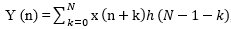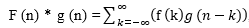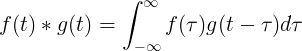код Convolution Calculator - Calculatorology

## Convolution Calculator

 Enter first data sequence: 1 1 1 0 0 0 Enter second data sequence: 0.5 0.2 0.3 Result data sequence:

### Convolution Calculator

The correlation function of f (T) is known as convolution and has the reversed function g(t-T). It is a calculator that is used to calculate a data sequence. It has two text fields where you enter the first data sequence and the second data sequence. The sequence of data entered in the text fields can be separated using spaces. The initial text field deals with whole numbers while the second is used to input the decimal data sequences.

The convolution calculator has two active controls that perform different functions. The ‘Calculate’ button is used to execute the calculations while the ‘Reset’ button is used to erase all data of the previous calculations from the text fields. The results of the data sequence is displayed in the bottom platform below the two controls of the calculator.

##### Formula
This calculator uses particular formula in performing the calculations., It means that the sequence y (n) is equivalent to the convolution of sequences h (n) and x (n)., it means that for finite sequences h (n) with N values and x (n) with M values.

For n = 0... M + N-2

##### Types of convolution
There are other types of convolution which utilize different formula in their calculations.

##### Discrete convolution, which is used to determine the convolution of two discrete functions.

##### Continuous convolution, which means that the convolution of g (t) and f (t) is equivalent to the integral of f(T) multiplied by f (t-T).

##### Convolution filter Implementation
Y (n) = x (n) * h (n). It means that the discrete input signal x (n) can be filtered by the convolution having the impulse response h (n) to derive the output signal y (n).